# Probabilistic Robotics Introduction Probabilities Bayes rule Bayes filters

• Slides: 35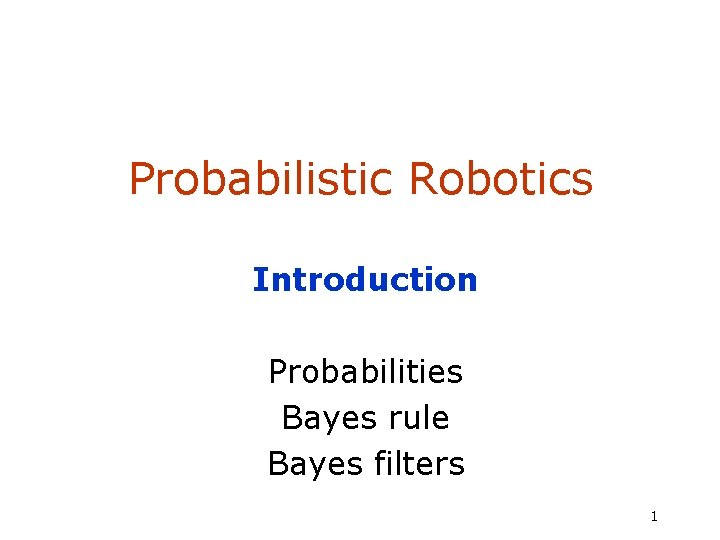Probabilistic Robotics Introduction Probabilities Bayes rule Bayes filters 1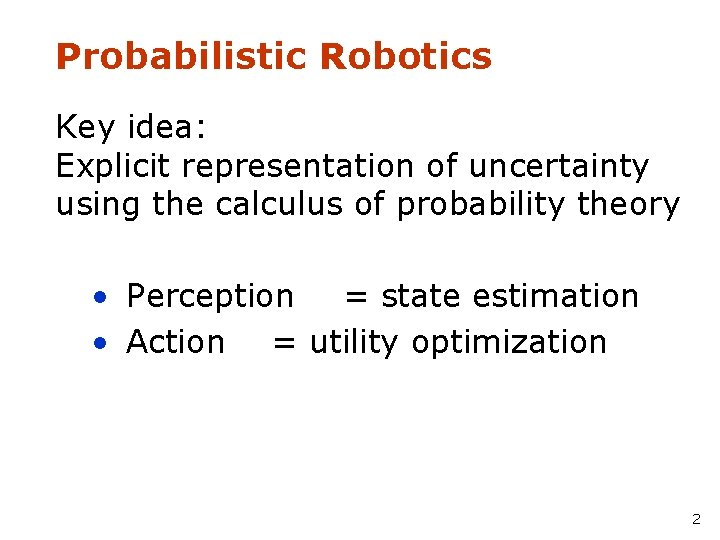Probabilistic Robotics Key idea: Explicit representation of uncertainty using the calculus of probability theory • Perception = state estimation • Action = utility optimization 2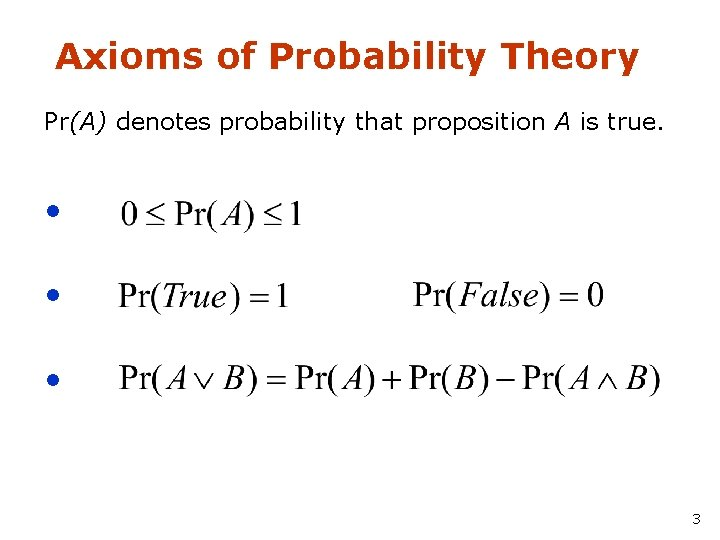Axioms of Probability Theory Pr(A) denotes probability that proposition A is true. • • • 3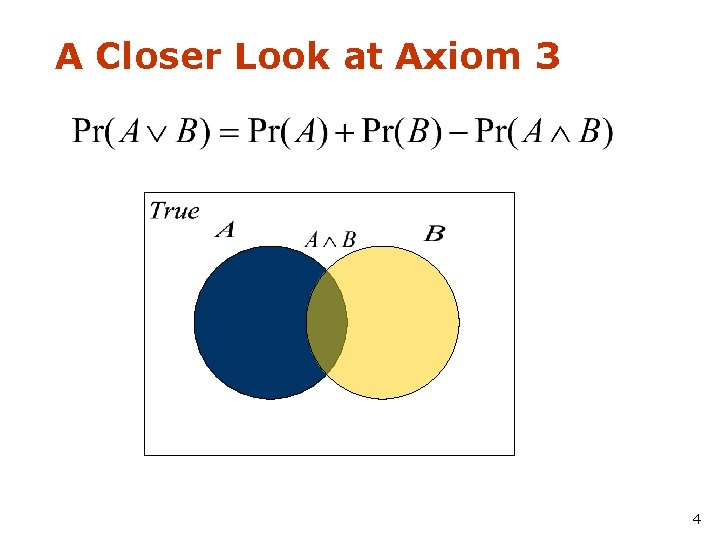A Closer Look at Axiom 3 B 4Using the Axioms 5Discrete Random Variables • X denotes a random variable. • X can take on a countable number of values in {x 1, x 2, …, xn}. • P(X=xi), or P(xi), is the probability that the random variable X takes on value. x i. • P( ) is called probability mass function. 6Continuous Random Variables • X takes on values in the continuum. • p(X=x), or p(x), is a probability density function. p(x) • E. g. x 7Joint and Conditional Probability • P(X=x and Y=y) = P(x, y) • If X and Y are independent then P(x, y) = P(x) P(y) • P(x | y) is the probability of x given y P(x | y) = P(x, y) / P(y) P(x, y) = P(x | y) P(y) • If X and Y are independent then P(x | y) = P(x) 8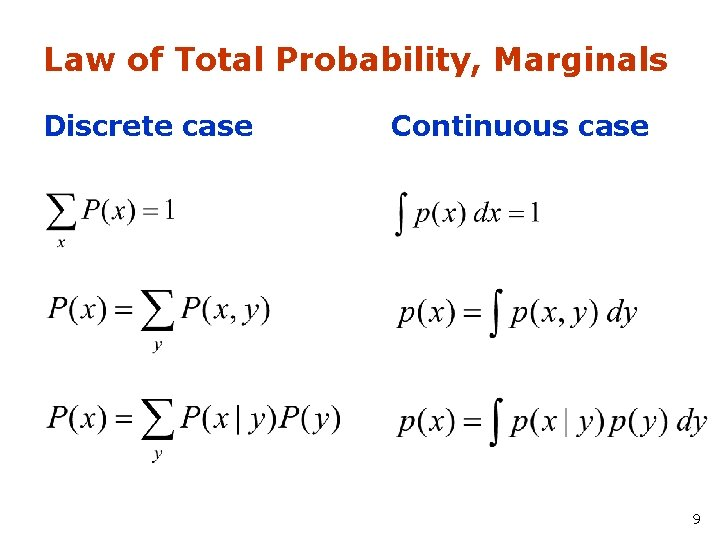Law of Total Probability, Marginals Discrete case Continuous case 9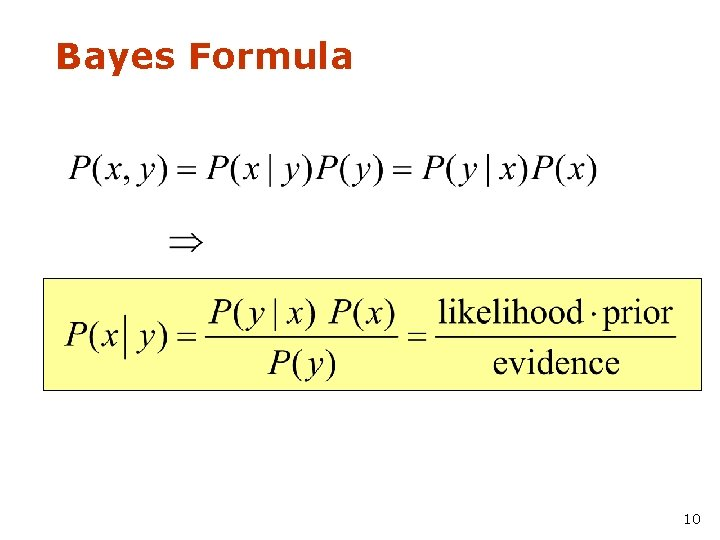Bayes Formula 10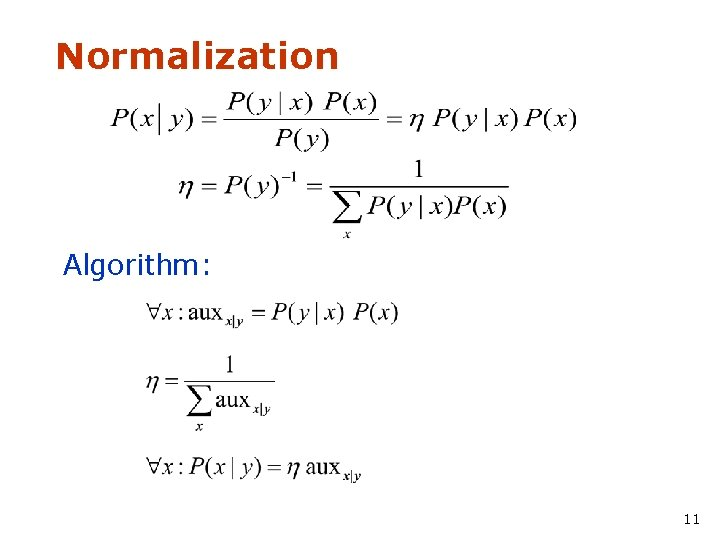Normalization Algorithm: 11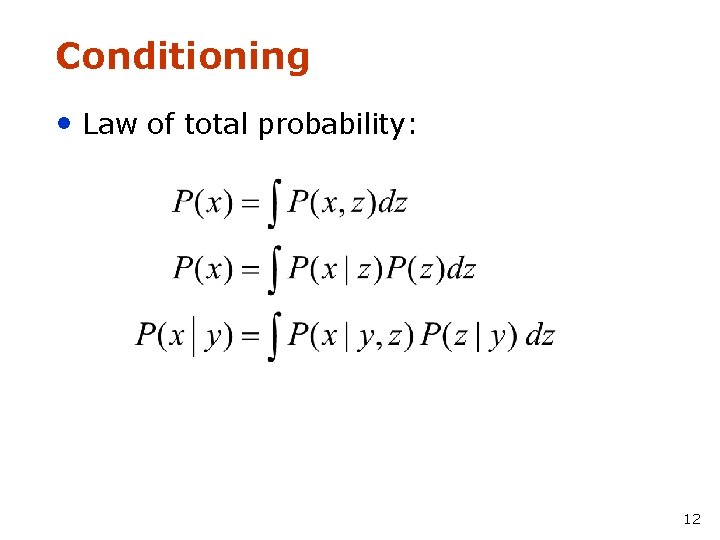Conditioning • Law of total probability: 12Bayes Rule with Background Knowledge 13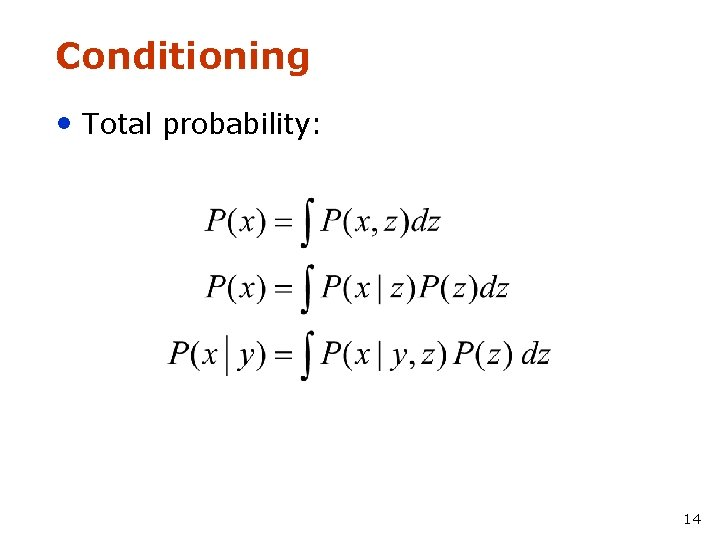Conditioning • Total probability: 14Conditional Independence equivalent to and 15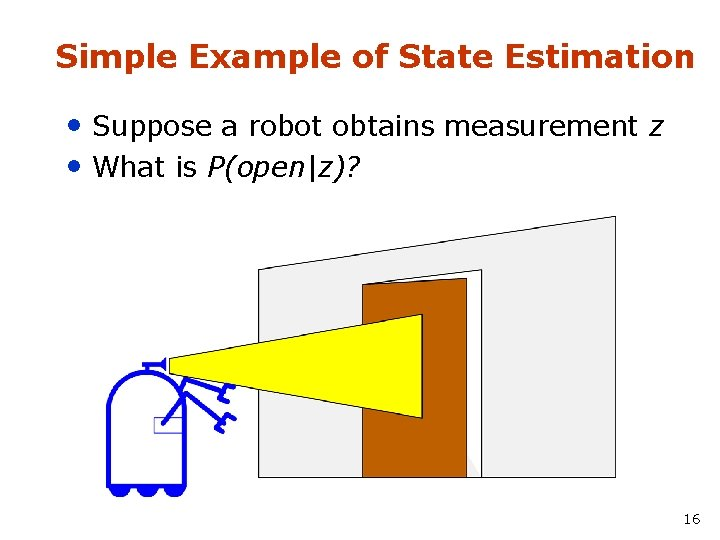Simple Example of State Estimation • Suppose a robot obtains measurement z • What is P(open|z)? 16Causal vs. Diagnostic Reasoning • P(open|z) is diagnostic. • P(z|open) is causal. • Often causal knowledge is easier to obtain. count frequencies! • Bayes rule allows us to use causal knowledge: 17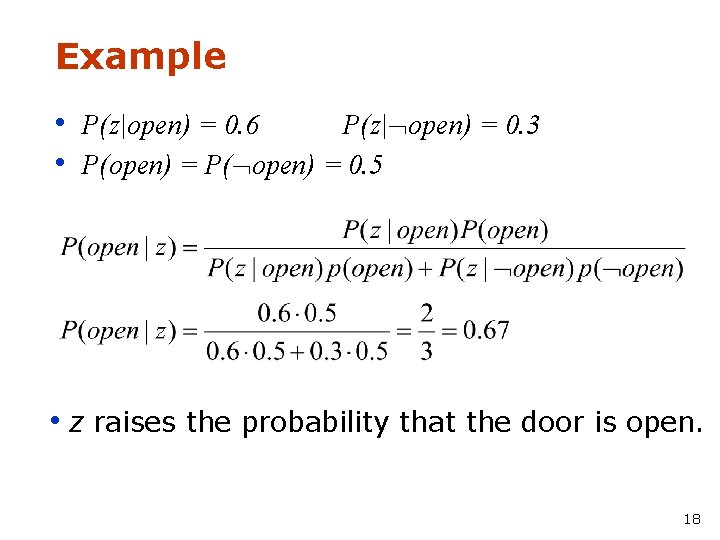Example • P(z|open) = 0. 6 P(z| open) = 0. 3 • P(open) = P( open) = 0. 5 • z raises the probability that the door is open. 18Combining Evidence • Suppose our robot obtains another observation z 2. • How can we integrate this new information? • More generally, how can we estimate P(x| z 1. . . zn )? 19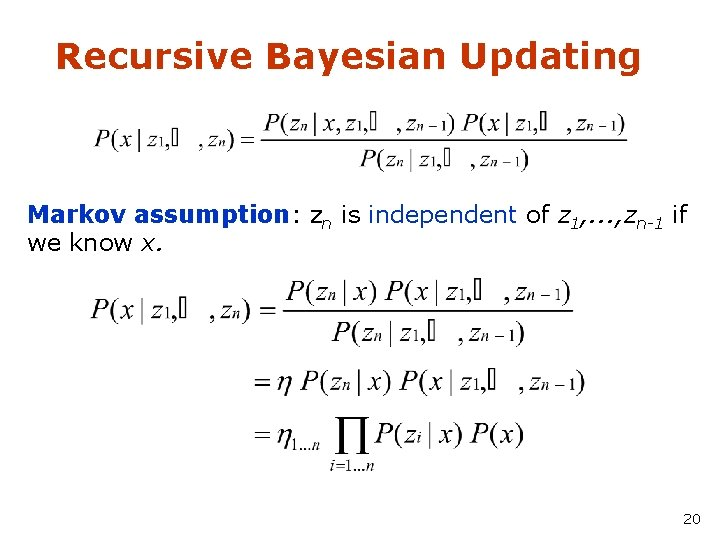Recursive Bayesian Updating Markov assumption: zn is independent of z 1, . . . , zn-1 if we know x. 20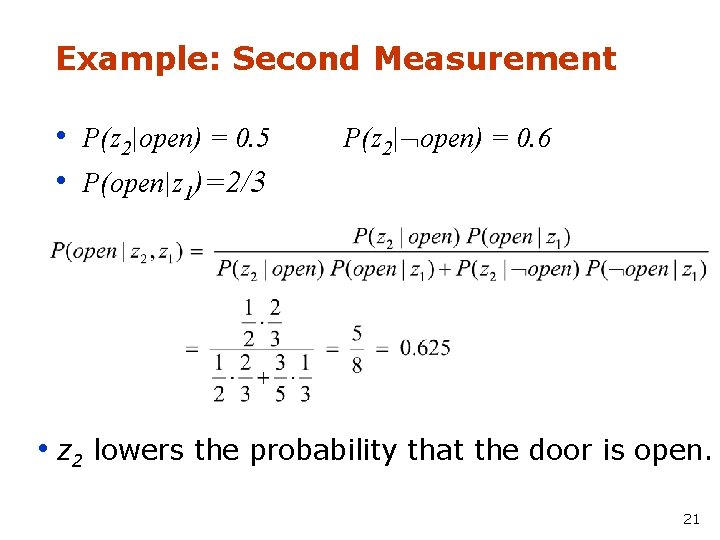Example: Second Measurement • P(z 2|open) = 0. 5 • P(open|z 1)=2/3 P(z 2| open) = 0. 6 • z 2 lowers the probability that the door is open. 21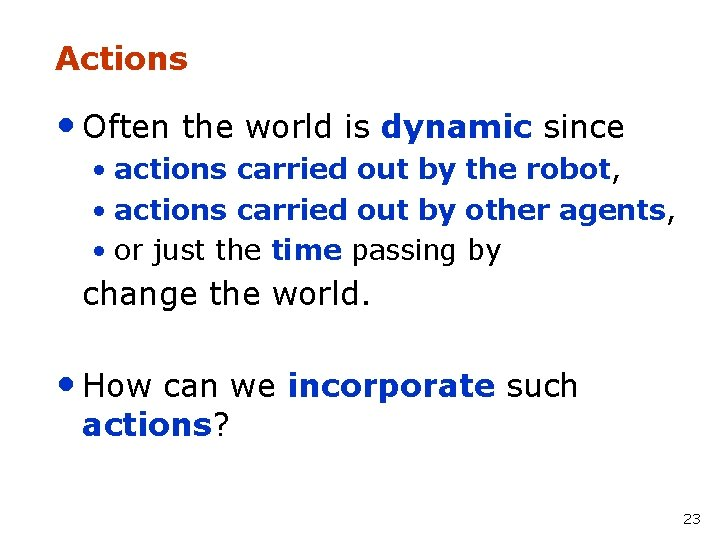Actions • Often the world is dynamic since • actions carried out by the robot, • actions carried out by other agents, • or just the time passing by change the world. • How can we incorporate such actions? 23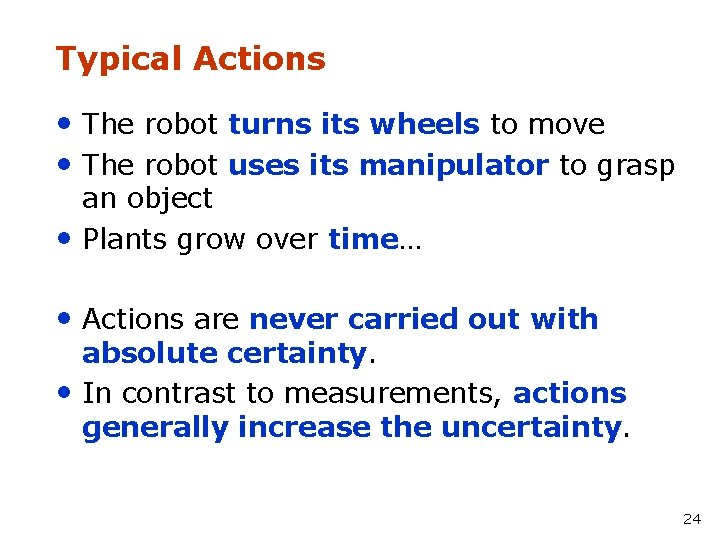Typical Actions • The robot turns its wheels to move • The robot uses its manipulator to grasp • an object Plants grow over time… • Actions are never carried out with • absolute certainty. In contrast to measurements, actions generally increase the uncertainty. 24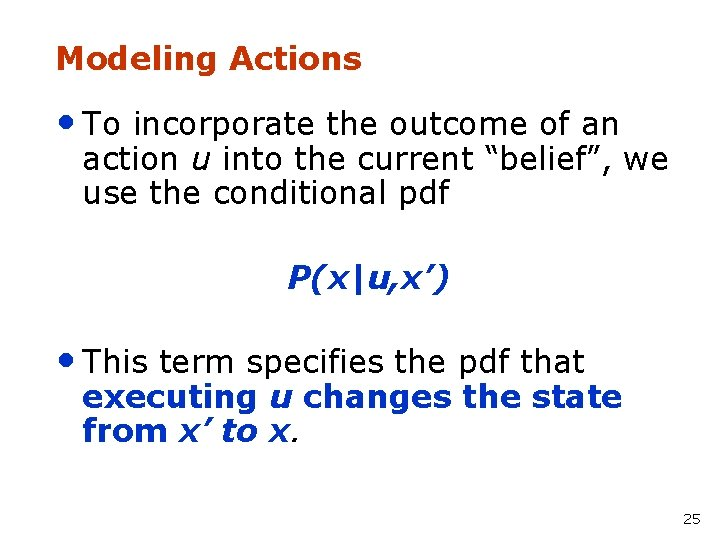Modeling Actions • To incorporate the outcome of an action u into the current “belief”, we use the conditional pdf P(x|u, x’) • This term specifies the pdf that executing u changes the state from x’ to x. 25Example: Closing the door 26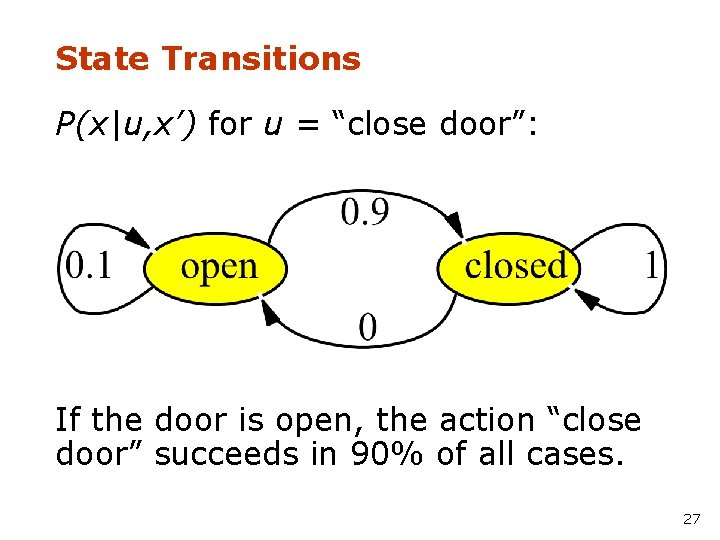State Transitions P(x|u, x’) for u = “close door”: If the door is open, the action “close door” succeeds in 90% of all cases. 27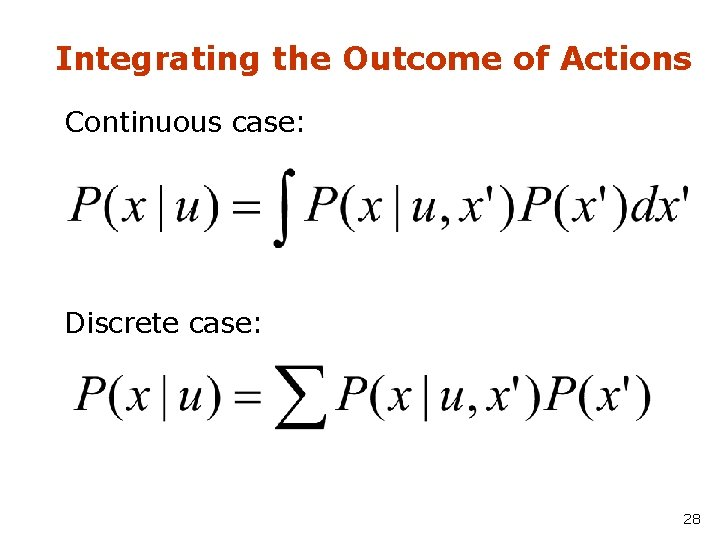Integrating the Outcome of Actions Continuous case: Discrete case: 28Example: The Resulting Belief 29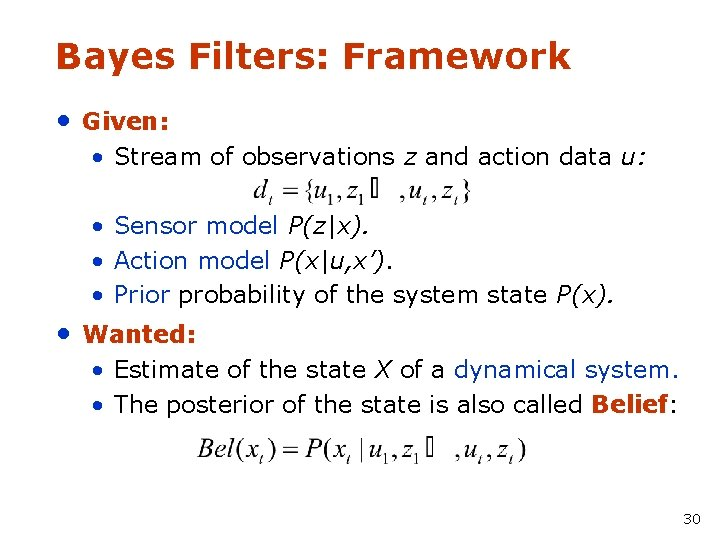Bayes Filters: Framework • Given: • Stream of observations z and action data u: • Sensor model P(z|x). • Action model P(x|u, x’). • Prior probability of the system state P(x). • Wanted: • Estimate of the state X of a dynamical system. • The posterior of the state is also called Belief: 30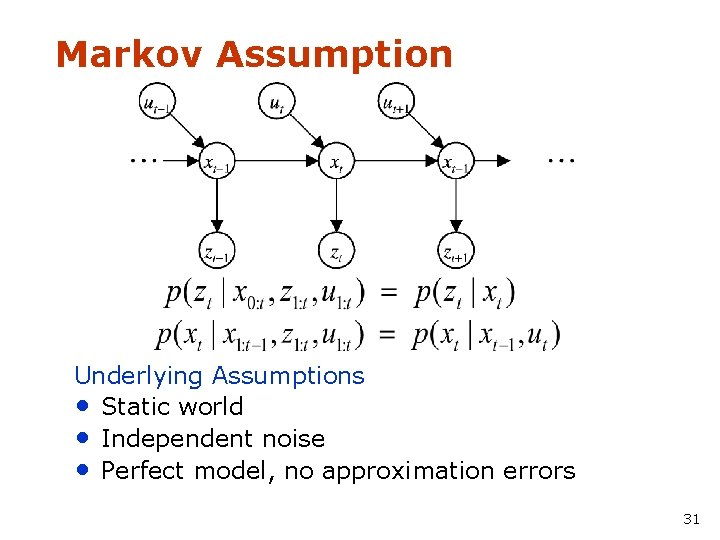Markov Assumption Underlying Assumptions • Static world • Independent noise • Perfect model, no approximation errors 31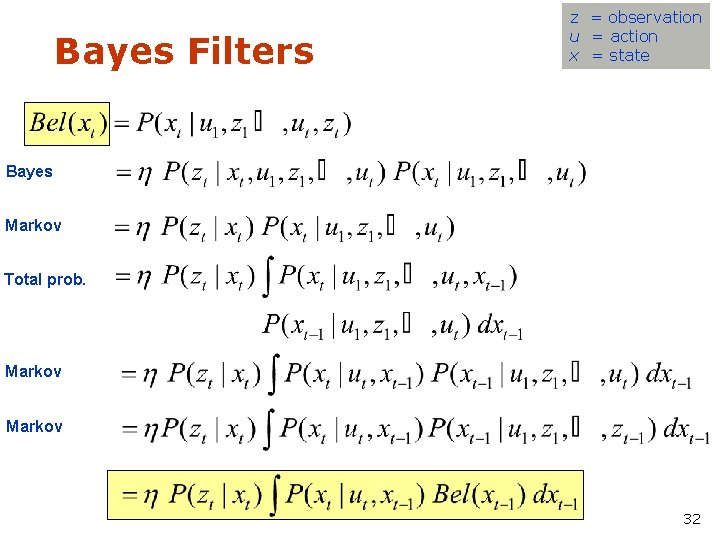Bayes Filters z = observation u = action x = state Bayes Markov Total prob. Markov 32Bayes Filter Algorithm 1. 2. Algorithm Bayes_filter( Bel(x), d ): h=0 3. 4. 5. 6. 7. 8. If d is a perceptual data item z then For all x do 9. Else if d is an action data item u then For all x do 10. 11. For all x do 12. Return Bel’(x) 33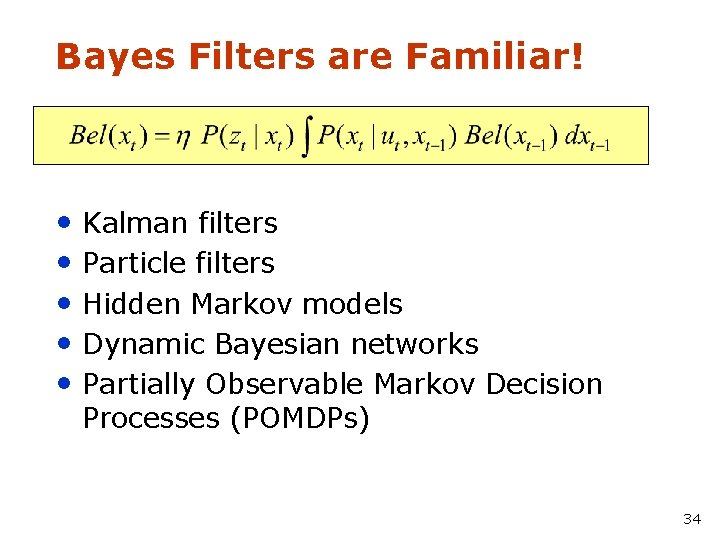Bayes Filters are Familiar! • Kalman filters • Particle filters • Hidden Markov models • Dynamic Bayesian networks • Partially Observable Markov Decision Processes (POMDPs) 34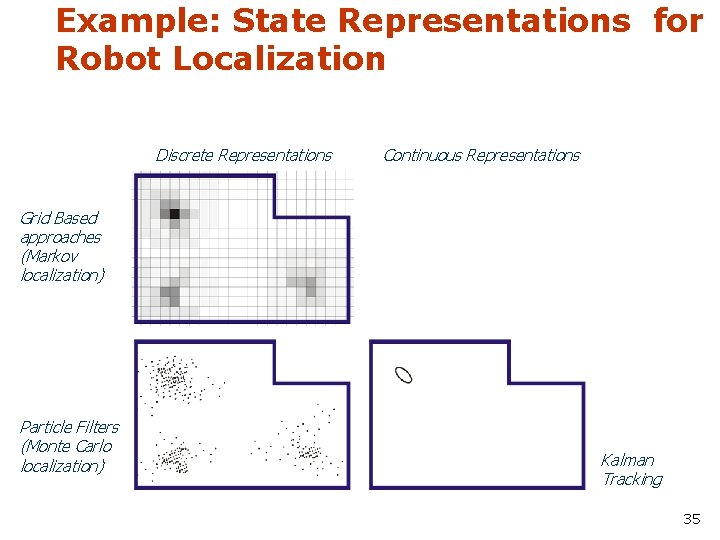Example: State Representations for Robot Localization Discrete Representations Continuous Representations Grid Based approaches (Markov localization) Particle Filters (Monte Carlo localization) Kalman Tracking 35Summary • Bayes rule allows us to compute probabilities that are hard to assess otherwise. • Under the Markov assumption, recursive Bayesian updating can be used to efficiently combine evidence. • Bayes filters are a probabilistic tool for estimating the state of dynamic systems. 36